# Trigonometry : Solving Triangles

## Example Questions

← Previous 1

### Example Question #1 : Finding Angles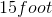plank has one end on the ground and one end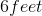off the ground. What is the measure of the angle formed by the plank and the ground?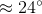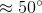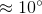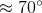Explanation:

The length of the plank becomes the hypotenuse of the triangle, while the distance between the plank and the ground becomes the length of one side. To solve for the angle between the plank and the ground, you must find the value of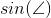. The sine of the angle is the value of the opposite side over the hypotenuse, which are values that we know.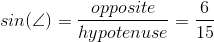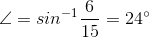### Example Question #2 : Finding Angles

Given that angle A is 23.0o, side a is 1.43 in., and side b is 3.62 in., what is the angle of B?

90o

81.5o

70o

83.6o

55o

81.5o

Explanation: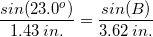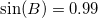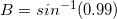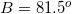### Example Question #331 : Trigonometry

Two angles in a triangle are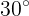and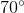. What is the measure of the 3rd angle?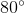There is not enough information to determine the angle measure.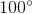Explanation:

The sum of the angles of a triangle is 180˚.

Thus, since the sum of our two angles is 100˚, our missing angle must be,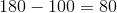### Example Question #1 : Finding Angles

If the hypotenuse of a right triangle has a length of 6, and the length of a leg is 2, what is the angle between the hypotenuse and the leg?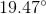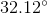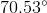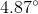Explanation:

The leg must be an adjacent side to the hypotenuse.

Therefore, we can use inverse cosine to solve for the angle.

First write the equation for sine of an angle.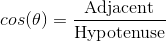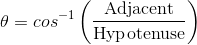Substitute the lengths given and solve for the angle.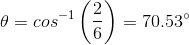### Example Question #1 : Finding Angles

A skateboard ramp made so that the rider can gain sufficient speed before a jump is 15 feet high and the ramp is 17 feet long. What is the measure of the angle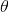between the ramp and the ground?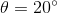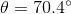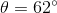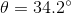Explanation:

For the angle in question we have the opposite side and the hypotenuse given to us. We can use the sine function.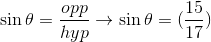Use the inverse sin to find the measure of an angle between these sides: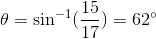### Example Question #6 : Finding Angles

What angle does the ramp make with the bottom of the stair?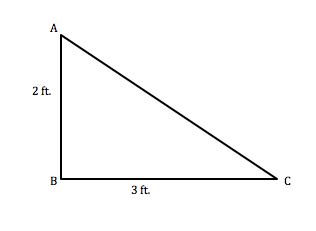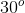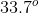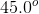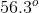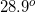Explanation: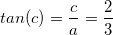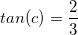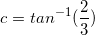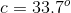### Example Question #111 : Triangles

If the height of the stair is 2 ft, and the length of the stair is 3 ft, how long must the ramp be to cover the stair?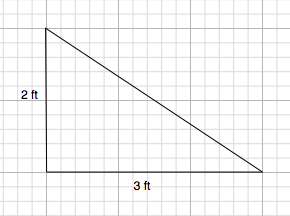13 ft

5 ft

2.5 ft

10 ft

3.6 ft

3.6 ft

Explanation:

Use the Pythagorean triangle to solve for the third side of the triangle.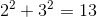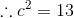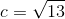Simplify and you have the answer: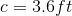### Example Question #112 : Triangles

A surveyor looks up to the top of a mountain at an angle of 35 degrees.  If the surveyor is 2400 feet from the base of the mountain, how tall is the mountain (to the nearest tenth of a foot)?

1966.0 feet

3427.6 feet

2135.8 feet

1680.5 feet

1376.6 feet

1680.5 feet

Explanation:

This is a typical angle of elevation problem.

From the question, we can infer that the ratio of the mountain height to the surveyor's horizontal distance from the mountain is equal to the tangent of 35 degrees - the mountain height is the "opposite side", while the horizontal distance is the "adjacent side".  In other words: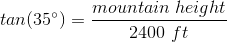Solving for the mountain height gives a vertical distance of 1680.5 feet.

### Example Question #111 : Triangles

In a right triangle, the legs areand. What is the length of the hypotenuse?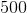Explanation:

Here, we can use the Pythagorean Theorem.

This states that the sum of the squares of the legs is equal to the square of the hypotenuse.

Thus, we plug into the formula to get our hypotenuse.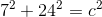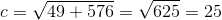### Example Question #114 : Triangles

If the altitude of a triangle has a height of 5, and also bisects a 60 degree angle, what must be the perimeter of the full triangle?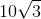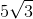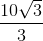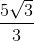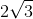Explanation:

The altitude of the triangle splits the 60 degree angle into two 30 degree angles.  Notice that this will create two right triangles with 30-60-90 angles.  This indicates that the full triangle is an equilateral triangle.

The altitude represents the adjacent side of the split triangles. The bisected angle is 30 degrees.  Find the hypotenuse of either split triangle.  This is the side length of the equilateral triangle.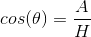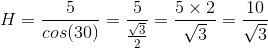Rationalize the denominator.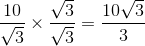Since the full triangle has three sides, multiply this value by three for the perimeter.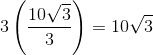← Previous 1

### All Trigonometry Resources